## Sunday, October 25, 2009

### C++ program to display a calender

A simple C++ graphics program to display a Calender.It also gives an introduction to mouse programming in graphics. Save the file with a '.cpp' extension and compile it.

#include<iostream.h>
#include<conio.h>
#include<graphics.h>
#include<stdlib.h>
#include<dos.h>
#include<string.h>
union REGS i,o;
initmouse();
showmouse();
hidemouse();
void restrictmouse(int x1,int y1,int x2,int y2);
void getmousestatus( int *button,int *x,int *y);
void findday();
void cal();
void main()
{
clrscr();
int gd=EGA,gm=EGAHI;
initgraph(&gd,&gm,"c:\\tc\\bgi ");
getch();
closegraph();
}
void findday()
{
int k=1,m=11,mon,D,C,f,i,y,total=0,t,I,d,x1=115,y1=160,q,r,v;
static int s=0;
char st2,st3,st4;
int days[]={31,28,31,30,31,30,31,31,30,31,30,31};
char
*month[]={"JANUARY","FEBRUARY","MARCH","APRIL","MAY","JUNE","JULY","AUGUST","SEPTEMBER","OCTOBER","NOVEMBER","DECEMEBER"};
restorecrtmode();
cout<<"Enter year : ";
cin>>y;
cout<<"Enter month : ";
cin>>mon;
if(mon>12)
{
cout<<" Invalid entry !.....";
delay(1000);
setgraphmode(getgraphmode());
}
y-=1;
C=y/100;
D=y%100;
f=(k+((13*m-1)/5)+D+(D/4)+(C/4)-(2*C));
i=f%7;
if(i< 0)
i+=7;
y++;
if(y%4==0)
days=29;
for(t=0;t<mon-1;t++)
{
total+=days[t];
}
I=total%7;
d=I+i;
if(d>=7)
d=d%7;
setgraphmode(getgraphmode());
cal();
v=mon-1;
strcpy(st3,month[v]);
itoa(y,st4,10);
settextstyle(2,0,8);
outtextxy(100,90,st3);
outtextxy(250,90,st4);
q=days[mon-1];
settextstyle(1,0,2);
setcolor(15);
for(r=1;r<=d;r++)
{
x1+=62;
s++;
}
for(r=1;r<=q;r++)
{
itoa(r,st2,10);
if(s>=6)
{
outtextxy(x1,y1,st2);
y1+=30;
x1=112;
s=0;
continue;
}
outtextxy(x1,y1,st2);
x1+=60;
s++;
}
s=0;
getch();
}
{
cleardevice();
int b,xx,yy;
initmouse();
restrictmouse(0,0,getmaxx(),getmaxy());
setbkcolor(0);
settextstyle(10,0,2);
outtextxy(100,150,"=> MONTH'S CALENDAR");
outtextxy(100,200,"=> EXIT");
settextstyle(8,0,1);
showmouse();
while(!kbhit())
{
getmousestatus(&b,&xx,&yy);
if(xx>=100&&yy>=150&&xx<=500&&yy<=200)
{
getmousestatus(&b,&xx,&yy);
if(b&1==1)
{
hidemouse();
cleardevice();
findday();
}
}
if(xx>=100&&yy>=200&&xx<=300&&yy<=260)
{
getmousestatus(&b,&xx,&yy);
if(b&1==1)
{
hidemouse();
cleardevice();
setbkcolor(0);
setcolor(15);
settextstyle(10,0,5);
outtextxy(100,200,"EXITING");
int o=0;
for(int n=0;n< 10;n++)
{
outtextxy(380+o,200,".");
o+=20;
delay(200);
}
exit(0);
}
}
}
}
void cal()
{
cleardevice();
int l=100,t=125,r=155,b=185,g,x=110,y=127;
char *day[]={"SUN","MON","TUE","WED","THU","FRI","SAT"};
char st1;
setbkcolor(0);
settextstyle(10,0,7);
setcolor(15);
outtextxy(100,-40,"C");
settextstyle(10,0,6);
outtextxy(160,-20,"ALENDAR");
setfillstyle(1,BLUE);
bar(85,115,530,345);
setfillstyle(1,0);
bar3d(l,t,r,b,0,0);
bar3d(l,t+30,r,b+30,0,0);
bar3d(l,t+60,r,b+60,0,0);
bar3d(l,t+90,r,b+90,0,0);
bar3d(l,t+120,r,b+120,0,0);
bar3d(l,t+150,r,b+150,0,0);
bar3d(l,t+180,r,b+150,0,0);
bar3d(l+60,t,r+60,b,0,0);
bar3d(l+60,t+30,r+60,b+30,0,0);
bar3d(l+60,t+60,r+60,b+60,0,0);
bar3d(l+60,t+90,r+60,b+90,0,0);
bar3d(l+60,t+120,r+60,b+120,0,0);
bar3d(l+60,t+150,r+60,b+150,0,0);
bar3d(l+60,t+180,r+60,b+150,0,0);
bar3d(l+120,t,r+120,b,0,0);
bar3d(l+120,t+30,r+120,b+30,0,0);
bar3d(l+120,t+60,r+120,b+60,0,0);
bar3d(l+120,t+90,r+120,b+90,0,0);
bar3d(l+120,t+120,r+120,b+120,0,0);
bar3d(l+120,t+150,r+120,b+150,0,0);
bar3d(l+120,t+180,r+120,b+150,0,0);
bar3d(l+180,t,r+180,b,0,0);
bar3d(l+180,t+30,r+180,b+30,0,0);
bar3d(l+180,t+60,r+180,b+60,0,0);
bar3d(l+180,t+90,r+180,b+90,0,0);
bar3d(l+180,t+120,r+180,b+120,0,0);
bar3d(l+180,t+150,r+180,b+150,0,0);
bar3d(l+180,t+180,r+180,b+150,0,0);
bar3d(l+240,t,r+240,b,0,0);
bar3d(l+240,t+30,r+240,b+30,0,0);
bar3d(l+240,t+60,r+240,b+60,0,0);
bar3d(l+240,t+90,r+240,b+90,0,0);
bar3d(l+240,t+120,r+240,b+120,0,0);
bar3d(l+240,t+150,r+240,b+150,0,0);
bar3d(l+240,t+180,r+240,b+150,0,0);
bar3d(l+300,t,r+300,b,0,0);
bar3d(l+300,t+30,r+300,b+30,0,0);
bar3d(l+300,t+60,r+300,b+60,0,0);
bar3d(l+300,t+90,r+300,b+90,0,0);
bar3d(l+300,t+120,r+300,b+120,0,0);
bar3d(l+300,t+150,r+300,b+150,0,0);
bar3d(l+300,t+180,r+300,b+150,0,0);
bar3d(l+360,t,r+360,b,0,0);
bar3d(l+360,t+30,r+360,b+30,0,0);
bar3d(l+360,t+60,r+360,b+60,0,0);
bar3d(l+360,t+90,r+360,b+90,0,0);
bar3d(l+360,t+120,r+360,b+120,0,0);
bar3d(l+360,t+150,r+360,b+150,0,0);
bar3d(l+360,t+180,r+360,b+150,0,0);
settextstyle(1,0,2);
setcolor(15);
for(g=0;g< 7;g++)
{
strcpy(st1,day[g]);
outtextxy(x,y,st1);
x+=60;
}
}
initmouse()
{
i.x.ax=0;
int86(0x33,&i,&o);
return(o.x.ax);
}
showmouse()
{
i.x.ax=1;
int86(0x33,&i,&o);
return(o.x.ax);
}
hidemouse()
{
i.x.ax=2;
int86(0x33,&i,&o);
return(o.x.ax);
}
void restrictmouse(int x1,int y1,int x2,int y2)
{
i.x.ax=7;
i.x.cx=x1;
i.x.dx=x2;
int86(0x33,&i,&o);
i.x.ax=8;
i.x.cx=y1;
i.x.dx=y2;
int86(0x33,&i,&o);
}
void getmousestatus(int *button,int *x,int *y)
{
i.x.ax=3;
int86(0x33,&i,&o);
*button=o.x.bx;
*x=o.x.cx;
*y=o.x.dx;
}

## Tuesday, October 13, 2009

### Simple C program for Scan Line Polygon Filling Algorithm

#include <stdio.h>
#include <conio.h>
#include <graphics.h>

main()
{

int n,i,j,k,gd,gm,dy,dx;
int x,y,temp;
int a,xi;
float slope;

clrscr();
printf("\n\n\tEnter the no. of edges of polygon : ");
scanf("%d",&n);
printf("\n\n\tEnter the cordinates of polygon :\n\n\n ");

for(i=0;i<n;i++)
{
printf("\tX%d Y%d : ",i,i);
scanf("%d %d",&a[i],&a[i]);
}

a[n]=a;
a[n]=a;

detectgraph(&gd,&gm);
initgraph(&gd,&gm,"c:\\tc\\bgi");

/*- draw polygon -*/

for(i=0;i<n;i++)
{
line(a[i],a[i],a[i+1],a[i+1]);
}

getch();

for(i=0;i<n;i++)
{
dy=a[i+1]-a[i];
dx=a[i+1]-a[i];

if(dy==0) slope[i]=1.0;
if(dx==0) slope[i]=0.0;

if((dy!=0)&&(dx!=0)) /*- calculate inverse slope -*/
{
slope[i]=(float) dx/dy;
}
}

for(y=0;y< 480;y++)
{
k=0;
for(i=0;i<n;i++)
{

if( ((a[i]<=y)&&(a[i+1]>y))||
((a[i]>y)&&(a[i+1]<=y)))
{
xi[k]=(int)(a[i]+slope[i]*(y-a[i]));
k++;
}
}

for(j=0;j<k-1;j++) /*- Arrange x-intersections in order -*/
for(i=0;i<k-1;i++)
{
if(xi[i]>xi[i+1])
{
temp=xi[i];
xi[i]=xi[i+1];
xi[i+1]=temp;
}
}

setcolor(35);
for(i=0;i<k;i+=2)
{
line(xi[i],y,xi[i+1]+1,y);
getch();
}

}

}

## Saturday, September 19, 2009

### Guide to prevent your computer from getting infected with Viruses that spread through removable media(like pen drives).

Most of us use removable media daily, for transferring data from one computer to another.We generally use pen drives with computers at offices, colleges, cyber cafes, etc...The computers at such places may or may not have anti-virus software installed. If the system you are using is infected with virus, then this virus will also get transferred onto your pen drive along with other data. If you use a pen drive containing virus, it will infect your system, which intern may lead to loss and/or theft of your personal data and leave you with no other option but to format your hard drive. Most common forms of such viruses are regsvr.exe,new folder.exe, etc..
I have been using this method to prevent my computer from getting infected with viruses,contained in removable media, for quiet some time now with great success, so I thought i'd share it with others.
All you need, to follow this guide is a good anti-virus software ( I recommend and use ESET NOD32 ).

STEP 1: Click 'start' and then select 'run'.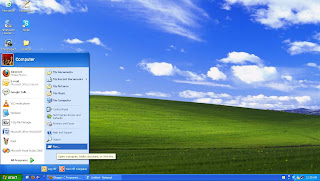STEP 2: In the dialogue box type 'gpedit.msc'.Doing this will give you access to the group policy editor.NOTE:- the group policy editor can only be accessed from the administrator's account.

SETP 3: A new window should now open. Click on 'Administrative templates'.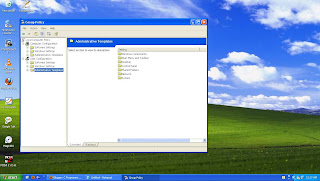STEP 4: Now on the left hand side, double click 'system'.This is what your window should look like.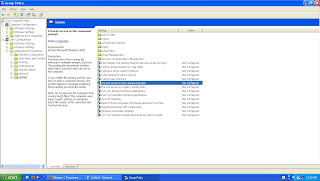STEP 7: Double click it and a new window should open.

STEP 8: Select 'enabled' and click 'apply'.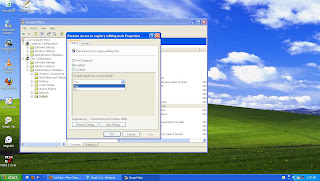This will prevent an unwanted application (Virus) to make any changes to the registry.

STEP 9: Now select 'turn off autoplay' from the list and double click it.STEP 10: Select 'enabled' and from the drop down box select 'all drives',click apply.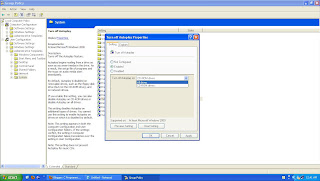This is what your window should look like:The virus contains an 'autorun.inf' file. Whenever you plug in your pen drive into the usb port an interrupt is generated,your computer services the interrupt with the appropriate ISR (interrupt service routine), this is how the computer (and the virus) know that some removable media has been connected to your computer. The autoplay feature reads this autorun.inf file and executes the commands listed in it. These commands are responsible for installing the virus files onto your system without your permission.So turning 'off' the autoplay wizard, does not allow the 'autorun.inf' to execute its code.

STEP 11: Now plug in the pen drive and go to 'my computer'.Step 12: 'Right click' on the icon that corresponds to your pen drive and select 'advanced options' and then 'clean files'.

STEP 13: Nod32 will now start scanning the removable media for viruses.

STEP 14: Once the scan is complete, select and delete all the infected files from the scan log.

STEP 15: Just to make sure that the autorun.inf file is no longer present on your pen drive, go to 'start',click run and type cmd (gives access to the command prompt). Type your drive letter followed by ':'. For eg. if my drive letter is 'i', I will type 'i:'. On the next line type 'dir'.This should give you a list of all the files present in your pen drive. If you see the 'autorun.inf' file in the list the simply delete it using this command 'del autorun.inf'.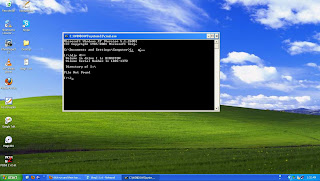STEP 16: The virus changes the attributes of all the folders on your pen drive to 'hidden', so to collectively remove the hidden attribute from all the files and view the files once again just type this in the command prompt:
I:\>attrib -H -S \*.* /S /D

STEP 17: Now open my computer and 'explore' (do not double click and access the drive as yet) your pen drive. At this point you should be able to see and access all files on your pen drive. Simply delete the files that you don't recognize or alternatively just copy all the required files onto some other location.

STEP 18: After all the required data as been recovered, format your pen drive !!

### C++ program to make a pie chart

#include<iostream.h>
#include<stdio.h>
#include<conio.h>
#include<math.h>
#include<graphics.h>
#include<dos.h>
#define round(a)(int(a+0.5))
void main()
{
double total=0.0,a=0.0;
double x2,y2;
int i,n;
int gd=DETECT,gm;
initgraph(&gd,&gm,"C:\\TC\\BGI");
cout<<"PIE CHART"<<endl;
cout<<"Enter the no. of regions"<<endl;
cin>>n;
double values;
double per;
double angle={0,0,0,0,0,0,0,0,0,0};
double b;
cout<<"Enter the values of the regions"<<endl;
circle(300,300,100);
line(300,300,400,300);
for(i=0;i<n;i++)
{
cin>>values[i];
total=total+values[i];
}
for(i=0;i<n;i++)
{
per[i]=((values[i]/total)*100);
a=((per[i]/100)*360);
if(i==0)
b[i]=a;
else
b[i]=b[i-1]+a;
angle[i]=(3.14*b[i])/180;
x2=(300+100*cos(angle[i]));
y2=(300-100*sin(angle[i]));
line(300,300,round(x2),round(y2));
setfillstyle(1,i+1);
if(x2>300&&y2< 300)
floodfill(x2+2,y2+2,15);
else
floodfill(x2-2,y2-2,15);
}
getch();
closegraph();
}